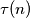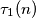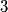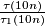### IMO Shortlist 2016 problem N2

Kvaliteta:
Avg: 0,0
Težina:
Avg: 6,0

Let$\tau(n)$ be the number of positive divisors of$n$. Let$\tau_1(n)$ be the number of positive divisors of$n$ which have remainders$1$ when divided by$3$. Find all positive integral values of the fraction$\frac{\tau(10n)}{\tau_1(10n)}$.

Izvor: https://www.imo-official.org/problems/IMO2016SL.pdf